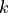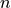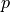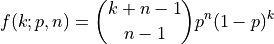# aesara.tensor.random.nbinom#

aesara.tensor.random.nbinom = <aesara.tensor.random.basic.NegBinomialRV object>[source]#

A negative binomial discrete random variable.

The probability mass function for `nbinom` for the numberof draws before observing theth success in terms of the probability of successof a single trial is: